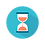From: Bayesian Models for Astrophysical Data, Cambridge Univ. Press

(c) 2017,  Joseph M. Hilbe, Rafael S. de Souza and Emille E. O. Ishida

you are kindly asked to include the complete citation if you used this material in a publication

Code 5.9  Log-inverse-Gaussian data

================================================

require(statmod)

set.seed(1056)                                                # set seed to replicate example
nobs = 3000                                                   # number of obs in model
x1 <- runif(nobs)                                           # random uniform variable
xb <- 1 + 0.5*x1
mu <- exp(xb)
y <- rinvgauss(nobs, mu, 20)                         # create y as adjusted random inverse-gaussian variate

================================================

Code 5.10  Log-inverse-Gaussian model in R using JAGS

================================================

require(R2jags)

X <- model.matrix(~ 1 + x1)
K <- ncol(X)

model.data <- list(Y = y,                               # response
X = X,                             # covariates
N = nobs,                        # sample size
K = K,                             # number of betas
Zeros = rep(0,nobs))

sink("IGGLM.txt")

cat("
model{
# Diffuse normal priors betas
for (i in 1:K) { beta[i] ~ dnorm(0, 0.0001)}

# Prior for lambda parameter of inverse Gaussian distribution
num ~ dnorm(0, 0.0016)                         # <----half-Cauchy(25)
denom ~ dnorm(0, 1)                              # <----half-Cauchy(25)
lambda <- abs(num / denom)                 # <----half-Cauchy(25)

# Likelihood
C <- 10000
for (i in 1:N){
Zeros[i] ~ dpois(Zeros.mean[i])
Zeros.mean[i] <- -L[i] + C
l1[i] <- 0.5 * (log(lambda) - log(2 * 3.141593 * Y[i]^3))
l2[i] <- -lambda * (Y[i] - mu[i])^2 / (2 * mu[i]^2 * Y[i])
L[i] <- l1[i] + l2[i]

log(mu[i]) <- inprod(beta[], X[i,])
}
}
",
fill = TRUE)

sink()

inits <- function () {
list(
beta = rnorm(ncol(X), 0, 0.1),
num = rnorm(1, 0, 25),
denom = rnorm(1, 0, 1) ) }

params <- c("beta", "lambda")

IVG <- jags(data = model.data,
inits = inits,
parameters = params,
model = "IGGLM.txt",
n.thin = 1,
n.chains = 3,
n.burnin = 2500,
n.iter = 5000)

print(IVG, intervals=c(0.025, 0.975), digits=3)

================================================Output on screen:

Inference for Bugs model at "IGGLM.txt", fit using jags,

3 chains, each with 5000 iterations (first 2500 discarded)

n.sims = 7500 iterations saved

mu.vect   sd.vect                    2.5%                   97.5%        Rhat        n.eff

beta                      0.995      0.015                   0.966                    1.023        1.001       4300

beta                      0.517      0.027                   0.467                    0.570        1.001       3500

lambda                    20.400     0.529                  19.374                  21.470        1.001       7500

deviance      60010045.450     2.579      60010042.573      60010052.445       1.000              1

For each parameter, n.eff is a crude measure of effective sample size,

and Rhat is the potential scale reduction factor (at convergence, Rhat=1).

DIC info (using the rule, pD = var(deviance)/2)

pD = 3.3 and DIC = 60010048.8

DIC is an estimate of expected predictive error (lower deviance is better).